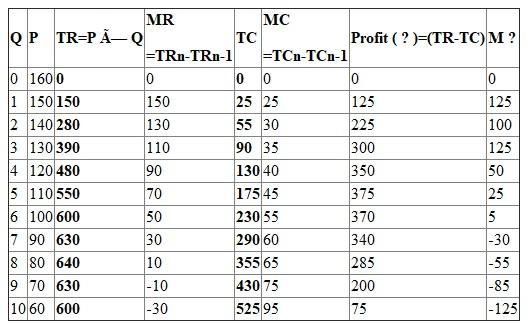## Quiz 2 : Economic OptimizationLooking for Economics Homework Help?# Quiz 2 : Economic Optimization

There is no answer for this question

Optimization means choosing the best alternative to achieve managerial goals. The decision of Chrysler Group to restructure its U.S. plant would be called partial optimization because the restructuring of plant would be carried only in one branch of the main company that is in U.S. The global optimization is the restructuring of whole company including all fields of activity including purchase, marketing, sale, human resource etc. Partial optimization on the other hand is the restructuring of a particular aspect of company.

a) The required table complete with formulas is below:b) The profit is maximized w hen Q=5. The maximum profit is \$ 375. c) The revenue (TR) is maximized at Q=8. The maximum revenue is \$640. d) The revenue maximizing output is generally greater than the profit maximizing output as profit is result of both total cost and total revenue. After some point, as the quantity increases, the increase in total revenue is less than increase in total cost. Hence the profit maximizing output is always less than the revenue maximizing output because cost is not considered in calculating revenues.

There is no answer for this question